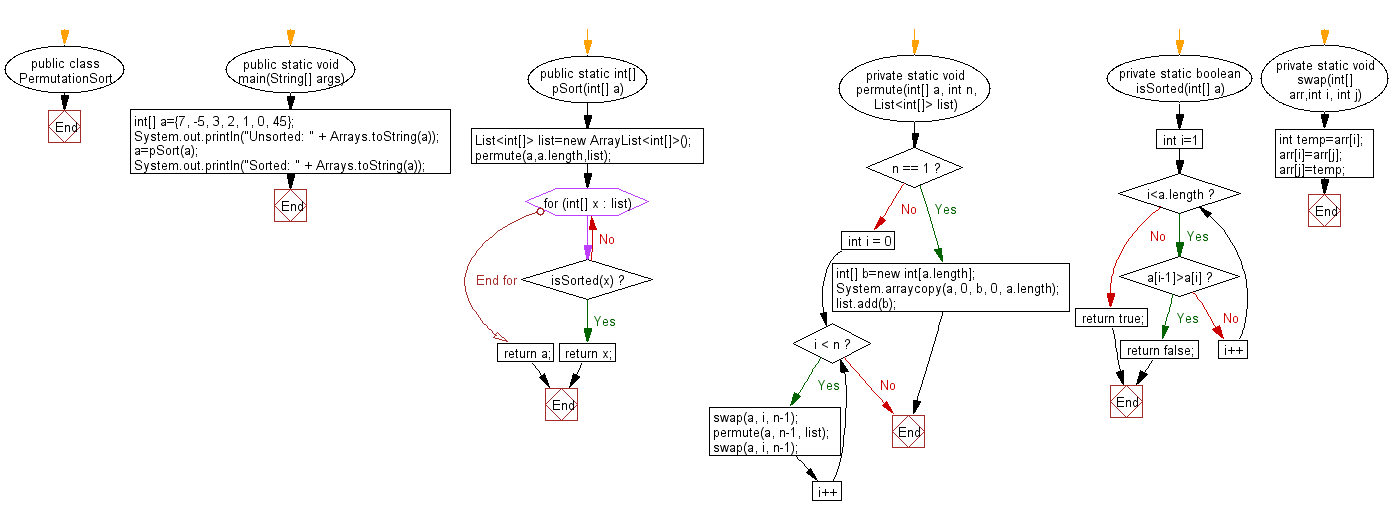﻿ Java exercises: Permutation sort Algorithm - w3resource# Java Exercises: Permutation sort Algorithm

## Java Sorting Algorithm: Exercise-15 with Solution

Write a Java program to sort an array of given integers using Permutation sort Algorithm.

Implement a permutation sort, which proceeds by generating the possible permutations of the input array/list until discovering the sorted one.

Sample Solution:

Java Code:

``````import java.util.List;
import java.util.ArrayList;
import java.util.Arrays;

public class PermutationSort
{
public static void main(String[] args)
{
int[] a={7, -5, 3, 2, 1, 0, 45};
System.out.println("Unsorted: " + Arrays.toString(a));
a=pSort(a);
System.out.println("Sorted: " + Arrays.toString(a));
}
public static int[] pSort(int[] a)
{
List<int[]> list=new ArrayList<int[]>();
permute(a,a.length,list);
for(int[] x : list)
if(isSorted(x))
return x;
return a;
}
private static void permute(int[] a, int n, List<int[]> list)
{
if (n == 1)
{
int[] b=new int[a.length];
System.arraycopy(a, 0, b, 0, a.length);
return;
}
for (int i = 0; i < n; i++)
{
swap(a, i, n-1);
permute(a, n-1, list);
swap(a, i, n-1);
}
}
private static boolean isSorted(int[] a)
{
for(int i=1;i<a.length;i++)
if(a[i-1]>a[i])
return false;
return true;
}
private static void swap(int[] arr,int i, int j)
{
int temp=arr[i];
arr[i]=arr[j];
arr[j]=temp;
}
}
```
```

Sample Output:

```Unsorted: [7, -5, 3, 2, 1, 0, 45]
Sorted: [-5, 0, 1, 2, 3, 7, 45]
```

Flowchart:Java Code Editor:

What is the difficulty level of this exercise?

﻿

## Java: Tips of the Day

Array vs ArrayLists:

The main difference between these two is that an Array is of fixed size so once you have created an Array you cannot change it but the ArrayList is not of fixed size. You can create instances of ArrayLists without specifying its size. So if you create such instances of an ArrayList without specifying its size Java will create an instance of an ArrayList of default size.

Once an ArrayList is full it re-sizes itself. In fact, an ArrayList is internally supported by an array. So when an ArrayList is resized it will slow down its performance a bit as the contents of the old Array must be copied to a new Array.

At the same time, it's compulsory to specify the size of an Array directly or indirectly while creating it. And also Arrays can store both primitives and objects while ArrayLists only can store objects.

Ref: https://bit.ly/3o8L2KH